# Compact space

## Definition

### Equivalent definitions in tabular format

No. Shorthand A topological space is said to be compact if ... A topological space$X$ is said to be compact if ...
1 Open cover-finite subcover formulation every open cover has a finite subcover for any collection$U_i, i \in I$ of open subsets of$X$ such that the union of the$U_i$s is$X$, there is a finite subset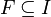$F \subseteq I$ such that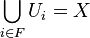$\bigcup_{i \in F} U_i = X$.
2 Finite intersection property formulation every family of closed sets with the finite intersection property has a nonempty overall intersection for any collection$C_i, i \in I$ of closed subsets of$X$ such that every intersection of finitely many of the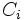$C_i$s is nonempty, we also have that the intersection of all$C_i$s is nonempty.
3 Ultrafilter formulation every ultrafilter of subsets converges to at least one point. if$\mathcal{F}$ is an ultrafilter of subsets of$X$, there exists$x \in X$ such that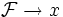$\mathcal{F} \to x$.
4 Subbasis open cover-finite subcover formulation (fix a choice of subbasis of open subsets) every open cover that uses only members of the subbasis has a finite subcover. (fix a choice of subbasis of open subsets) for any collection$U_i, i \in I$ of open subsets of$X$, all from within the subbasis, such that the union of the$U_i$s is$X$, there is a finite subset$F \subseteq I$ such that$\bigcup_{i \in F} U_i = X$.

### Equivalence of definitions

The equivalence with definition (4) follows from the Alexander subbase theorem.

## Examples

### In the real line and Euclidean space

• Any interval of the form$[a,b]$ (with both$a$ and$b$ real numbers) is a compact space, with the subspace topology inherited from the usual topology on the real line. More generally, any finite union of such intervals is compact.
• Compact subsets could look very different from unions of intervals. For instance, the Cantor set is compact.
• A subset of the real line, or more generally, of Euclidean space, is compact with the subspace topology if and only if it is closed and bounded (i.e., it can be enclosed inside some large enough ball). See Heine-Borel theorem
• Note that it is not true for arbitrary metric spaces that closed and bounded subsets are compact. In fact, for normed real and complex vector spaces, that occur extensively in functional analysis, closed and bounded iff compact is equivalent to being finite-dimensional. Much of the difficulty and challenge of dealing with infinite-dimensional normed real and complex vector spaces is coming up with conditions analogous to compactness that allow reasoning similar to that done in the finite-dimensional case.

### More general examples

• For a metric space to be compact with the induced topology is equivalent to a condition on it called being totally bounded. See compact metric space.
• The geometric realization of any finite simplicial complex is a compact space. (Geometric realizations of simplicial complexes are called polyhedra). See compact polyhedron.
• The geometric realiation of a CW-complex with finitely many cells is a compact space. (Geometric realizations of CW-complexes are termed CW-spaces).
• Some (but not all) manifolds are compact manifolds, and much of the theory of manifolds relies on the crucial distinction between compact and non-compact manifolds.

### In commutative algebra

The spectrum of a commutative unital ring, equipped with the Zariski topology, is always compact (though almost never Hausdorff).

This article defines a property of topological space that is pivotal (viz important) among currently studied properties of topological spaces

View a complete list of basic definitions in topology

## Relation with other properties

This property is a pivotal (important) member of its property space. Its variations, opposites, and other properties related to it and defined using it are often studied

### Stronger properties

Property Meaning Proof of implication Proof of strictness (reverse implication failure) Intermediate notions
compact Hausdorff space compact and Hausdorff: distinct points are separated by disjoint open subsets Compact T1 space|FULL LIST, MORE INFO
compact metrizable space compact and metrizable: arises from a metric space Compact Hausdorff space|FULL LIST, MORE INFO
compact manifold compact and a manifold
compact polyhedron compact and a polyhedron: arises from a simplicial complex
supercompact space there is a subbasis of open subsets such that every open cover using the subbasis has a subcover using at most two subsets |FULL LIST, MORE INFO
Noetherian space descending chain of closed subsets stabilizes in finitely many steps Noetherian implies compact compact not implies Noetherian |FULL LIST, MORE INFO
hereditarily compact space every subspace is compact compactness is not hereditary |FULL LIST, MORE INFO

### Weaker properties

Property Meaning Proof of implication Proof of strictness (reverse implication failure) Intermediate notions
locally compact space every point is contained in an open subset that's contained in a closed compact subset compact implies locally compact locally compact not implies compact |FULL LIST, MORE INFO
paracompact space every open cover has a locally finite open refinement compact implies paracompact paracompact not implies compact |FULL LIST, MORE INFO
limit point-compact space every infinite set has a limit point compact implies limit point-compact limit point-compact not implies compact |FULL LIST, MORE INFO
countably compact space every countable open cover has a finite subcover compact implies countably compact countably compact not implies compact |FULL LIST, MORE INFO
sequentially compact space every infinite sequence has a convergent subsequence compact implies sequentially compact sequentially compact not implies compact |FULL LIST, MORE INFO
Lindelof space every open cover has a countable subcover compact implies Lindelof Lindelof not implies compact |FULL LIST, MORE INFO

## Metaproperties

Metaproperty name Satisfied? Proof Statement with symbols
product-closed property of topological spaces Yes Tychonoff theorem (for arbitrary products). This requires the axiom of choice. For products of finitely many spaces, the proof is simpler and can be deduced from the tube lemma. If$X_i, i \in I$, are all compact spaces, then the product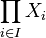$\prod_{i \in I} X_i$, endowed with the product topology, is also a compact space.
Version for two spaces: If$X$ and$Y$ are compact, the product space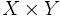$X \times Y$ with the product topology is also compact.
weakly hereditary property of topological spaces Yes Compactness is weakly hereditary If$X$ is a compact space and$A$ is a closed subset of$X$, then$A$ is a compact space with the subspace topology.
subspace-hereditary property of topological spaces No Compactness is not subspace-hereditary It is possible to have a compact space$X$ and a subset$A$ of$X$ such that$A$ is not a compact space with the subspace topology.
fiber-bundle-closed property of topological spaces Yes Compactness is fiber bundle-closed If$p:E \to B$ is a fiber bundle with fiber space$F$, and both$B$ and$F$ are compact, then$E$ is compact.
continuous image-closed property of topological spaces Yes Compactness is continuous image-closed If$f:X \to Y$ is a continuous map of topological spaces and$X$ is a compact space, then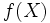$f(X)$ is a compact space under the subspace topology from$Y$. In particular, if$f$ is surjective, then$Y$ is compact.
coarsening-preserved property of topological spaces Yes Compactness is coarsening-preserved If a set$X$ is compact under a particular topology, it is also compact under any coarser topology.

## Formalisms

### Refinement formal expression

In the refinement formalism, the property of compactness has the following refinement formal expression:

Open$\to$ Finite open